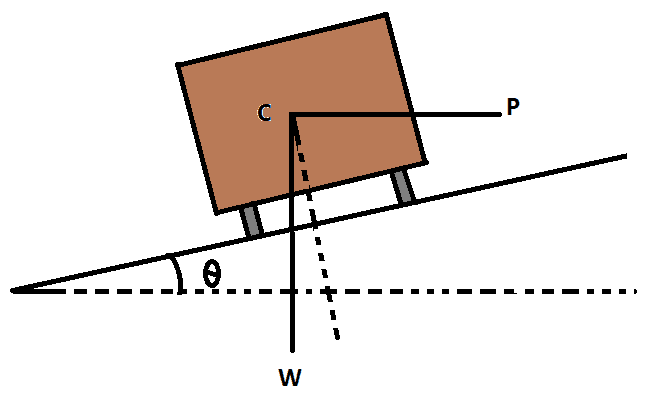# Analysis of Superelevation Formula as Per I.R.C

27th March 2019

## Analysis of Superelevation

Let, W = Weight of the vehicle,
v = Speed of the vehicle in m/sec,
g = Acceleration due to gravity in m/sec²,
f = Coefficient of friction,
e = rate of superelevation (tanθ),
F = Frictional force resistance due to centrifugal force,
P = Centrifugal force.

Now, P = Wv²/gR

For equilibrium, resolving the forces parallel to the inclined plane, are
P Cosθ = W Sinθ + F
Or, P Cosθ = W Sinθ + f(W Cosθ + P Sinθ)

After putting the value of P(P = Wv²/gR) we get,
Wv² Cosθ / gR = W Sinθ + f(W Cosθ + Wv² Sinθ / gR)
Or, v² Cosθ / gR = Sinθ + f(Cosθ + v² Sinθ / gR)

Now, both side divided by Cosθ, After that we get the following equation:
v² / gR = tanθ + f(1 + v² tanθ / gR)
Or, v² / gR = tanθ + f + fv² tanθ / gR

The term fv² tanθ / gR being too small and can be neglected.
Now,
v² / gR = tanθ + f
Or, v² / gR = e + f  …………(1)

### Formula of Superelevation

If the speed of the vehicle is given as V Km/h, then

v = V⨯1000 / 60⨯60 m/Sec Or, v = 0.278V

Then the equation (1) becomes  (0.278V)² / 9.81 R = e + f

Or, e + f = V² / 127R

### Formula Of equilibrium Superelevation

If we provide e = V² / 127R, then the value of ‘f’ will be equal to zero and the lateral friction will not be considered. This superelevation is called equilibrium superelevation.

Equilibrium superelevation (e) = V² / 127R

### Design Formula of Superelevation as Per IRC

As per I.R.C, superelevation is calculated at three fourth the design speed

e = (0.75V)² / 127R. Or, e = V² / 225R